###### Please wait...
Contact Us
Contact
Need assistance? Contact us on below numbers

For Study plan details

10:00 AM to 7:00 PM IST all days.

Franchisee/Partner Enquiry (North)

Franchisee/Partner Enquiry (South)

Franchisee/Partner Enquiry (West & East)

OR

or

Thanks, You will receive a call shortly.
Customer Support

You are very important to us

For any content/service related issues please contact on this number

8788563422

Mon to Sat - 10 AM to 7 PM

# RD Sharma Solution for Class 12 Humanities Mathematics Chapter 1 - Relations

Share this:
Exercise/Page

## RD Sharma Solution for Class 12 Humanities Mathematics Chapter 1 - Relations Page/Excercise 1.1

Solution 1(i)Solution 1(ii)Solution 1(iii)Solution 1(iv)Solution 2Solution 3(i)Solution 3(ii)Solution 3(iii)Solution 4Solution 5(i)Solution 5(ii)Solution 5(iii)Solution 6Solution 7Solution 8Solution 9Solution 10Solution 11Solution 12Solution 13Solution 14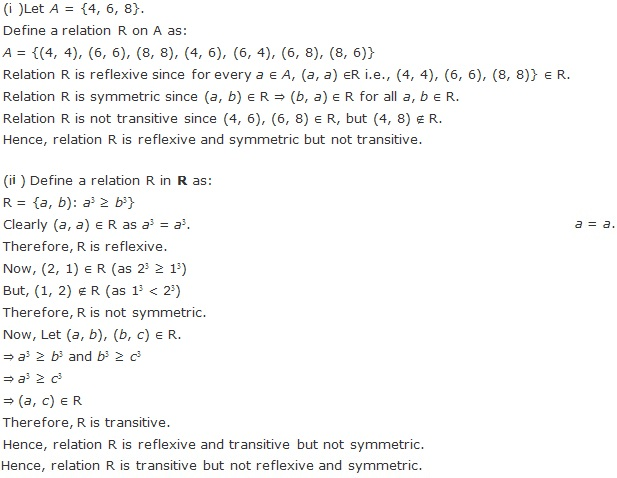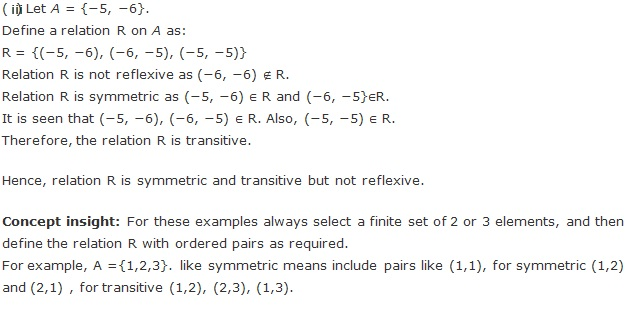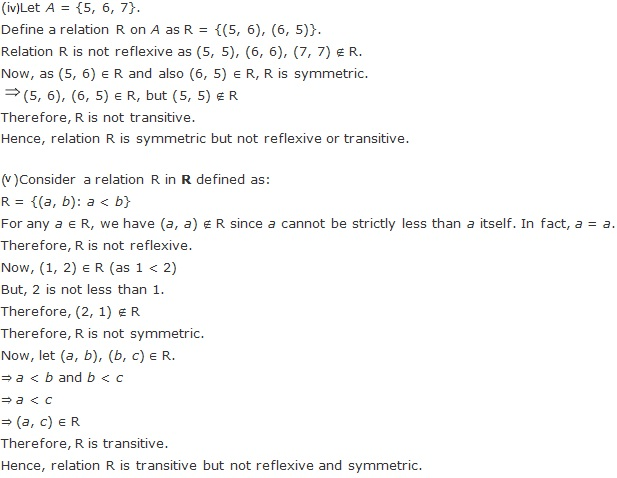Solution 15Solution 16Solution 17

A relation R in A is said to be reflexive if aRa for all aA

R is said to be transitive if aRb and bRc aRc

for all a, b, c A.

Hence for R to be reflexive (b, b) and (c, c) must be there in the set R.

Also for R to be transitive (a, c) must be in R because (a, b) R and (b, c) R so (a, c) must be in R.

So at least 3 ordered pairs must be added for R to be reflexive and transitive.

Solution 18

A relation R in A is said to be reflexive if aRa for all aA, R is symmetric if aRb bRa, for all a, b A and it is said to be transitive if aRb and bRc aRc for all a, b, c A.

x > y,  x, y ϵ N

(x, y) ϵ {(2, 1), (3, 1).......(3, 2), (4, 2)....}

This is not reflexive as (1, 1), (2, 2)....are absent.

This is not symmetric as (2,1) is present but (1,2) is absent.

This is transitive as (3, 2) ϵ R and (2,1) ϵ R also (3,1) ϵ R ,similarly this property satisfies all cases.

x + y = 10,  x, y ϵ N

(x, y)ϵ {(1, 9), (9, 1), (2, 8), (8, 2), (3, 7), (7, 3), (4, 6), (6, 4), (5, 5)}

This is not reflexive as (1, 1),(2, 2)..... are absent.

This only follows the condition of symmetric set as  (1, 9)ϵR also (9, 1)ϵR similarly other cases are also satisfy the condition.

This is not transitive because {(1, 9),(9, 1)}ϵR but (1, 1) is absent.

xy is square of an integer, x, y ϵ N

(x, y) ϵ {(1, 1), (2, 2), (4, 1), (1, 4), (3, 3), (9, 1),  (1, 9), (4, 4), (2, 8), (8, 2), (16, 1), (1, 16)...........}

This is reflexive as (1,1),(2,2)..... are present.

This is also symmetric because if aRb bRa, for all a,bϵN.

This is transitive also because if aRb and bRc aRc for all a, b, c ϵ N.

x + 4y = 10, x, y ϵ N

(x, y) ϵ {(6, 1), (2, 2)}

This is not reflexive as (1, 1), (2, 2).....are absent.

This is not symmetric because (6,1) ϵ R but (1,6) is absent.

This is not transitive as there are only two elements in the set having no element common.

## RD Sharma Solution for Class 12 Humanities Mathematics Chapter 1 - Relations Page/Excercise 1.2

Solution 1Solution 2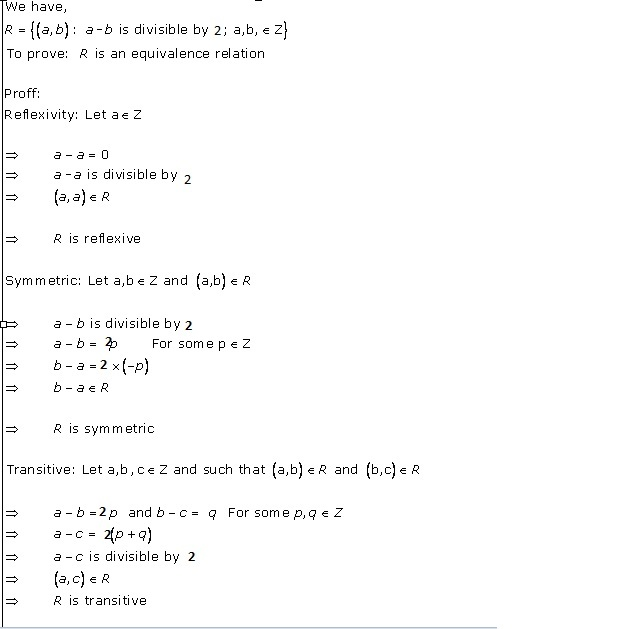Solution 3Solution 4Solution 5Solution 6Solution 7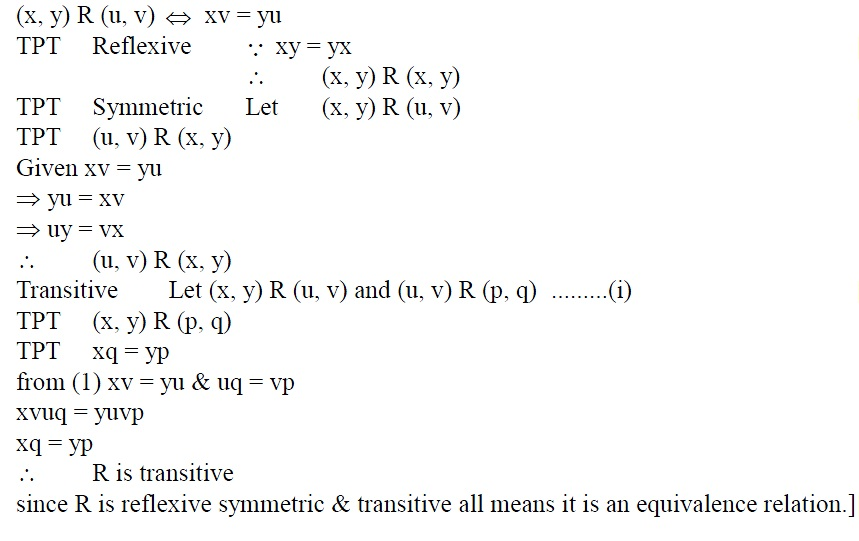Solution 8Also we need to find the set of all elements related to 1.

Since the relation is given by, R={(a,b):a=b}, and 1 is an element of A,

R={(1,1):1=1}

Thus, the set of all elements related to 1 is 1.

Solution 9Solution 10Solution 11Solution 12Solution 13Solution 14Solution 15Solution 16## RD Sharma Solution for Class 12 Humanities Mathematics Chapter 1 - Relations Page/Excercise MCQ

Solution 1Solution 2Solution 3Solution 4Solution 5Solution 6Solution 7Solution 8Solution 9Solution 10Solution 11Solution 12Solution 13Solution 14Solution 15Solution 16Solution 17Solution 18Solution 19Solution 20Solution 21Solution 22Solution 23Solution 24Solution 25Solution 26Solution 27Solution 29